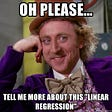# What is Confusion Matrix?

• The target variable has two values: Positive or Negative
• The columns represent the actual values of the target variable
• The rows represent the predicted values of the target variable

# Understanding True Positive, True Negative, False Positive, and False Negative in a Confusion Matrix

• The predicted value matches the actual value
• The actual value was positive and the model predicted a positive value
• The predicted value matches the actual value
• The actual value was negative and the model predicted a negative value
• The predicted value was falsely predicted
• The actual value was negative but the model predicted a positive value
• Also known as the Type 1 error
• The predicted value was falsely predicted
• The actual value was positive but the model predicted a negative value
• Also known as the Type 2 error
• True Positive (TP) = 560; meaning 560 positive class data points were correctly classified by the model
• True Negative (TN) = 330; meaning 330 negative class data points were correctly classified by the model
• False Positive (FP) = 60; meaning 60 negative class data points were incorrectly classified as belonging to the positive class by the model
• False Negative (FN) = 50; meaning 50 positive class data points were incorrectly classified as belonging to the negative class by the model

# Why does Confusion Matrix need to use evaluate IDS?

--

--

--

## More from amit soni

Love podcasts or audiobooks? Learn on the go with our new app.

## Easy pivot() in BigQuery, finally## IBM Cloud Pak for Data — using Decision Optimization## Train, Evaluate, Visualize with One Line of Code## Data-Science Series (Part 6)-Discretization, Continuation, Normalization, Randomization on the data…## Linear Regression : All possible techniques you need to know## The DAP Journey: Python analysis of gerrymandering## 10 Techniques to deal with Imbalanced Classes in Machine Learning!## LeetCode 206. Reverse a Linked List